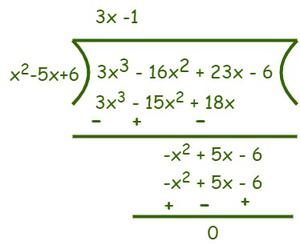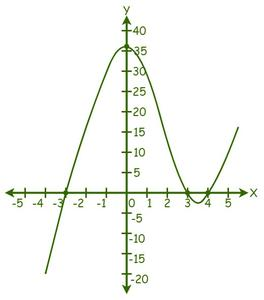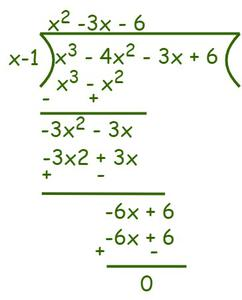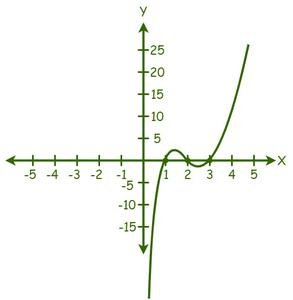Open in App
Not now

# Solving Cubic Equations – Methods and Examples

• Last Updated : 31 Jan, 2023

In mathematics, an algebraic expression that consists of variables, coefficients, and arithmetic operations such as addition, subtraction, multiplication, or division is called a polynomial. The general form of a polynomial is axn + bxn-1 + cxn-2 +… + 1. An equation is a mathematical statement expressing the relationship between two values. An algebraic equation is an equation that is in the form of axn + bxn-1 + cxn-2 +… + 1 = 0. For example, 2x-5 = 0 is an example of an algebraic equation where (2x-5) is a polynomial. There are various types of algebraic equations depending upon the highest degree of the variable such as a linear equation, quadratic equation, cubic, equation, etc.

Cubic equation is an algebraic equation where the highest degree of the polynomial is 3. Some examples of cubic equations are

x3 – 4x2 + 15x – 9 = 0, 2x3 – 4x2 + 5 = 0, etc.

The general form of a cubic equation is,

ax3 + bx2 + cx + d = 0, a ≠ 0

where,

a, b, and c are the coefficients and d is the constant.

### How to solve Cubic Equations?

A cubic equation can be solved traditionally by reducing it into a quadratic equation and then solving it either by factoring or the quadratic formula. Like a quadratic equation has two roots, a cubic equation has three roots. A cubic equation may have three real roots or a real root and two imaginary roots. Any equation, including cubic equations, must always be arranged in its standard form first.

For instance, if the given equation is 2x2-5 = x + 4/x, then we have to re-arrange this into its standard form, i.e., 2x3-x2-5x-4 = 0. Now, we can solve the equation using any appropriate method.

A cubic equation can be solved in the following ways:

• Finding Integer Solutions with Factor Lists
• Using the Graphical method

### Solving the cubic equation using factors of the polynomial

Example:  Find the roots of equation f(x) = 3x3 −16x2 + 23x − 6 = 0.

Solution:

Given expression: f(x) = 3x3 −16x2 + 23x − 6 = 0.

First, factorize the polynomial to get roots.

Since the constant is +6 the possible factors are 1, 2, 3, 6.

f(1) = 3 – 16 + 23 – 6 ≠ 0

f(2) = 24 – 64 + 46 – 6 = 0

f(3) = 81 – 144 + 69 – 6 = 0

f(6) = 648 – 576 + 138 – 6 ≠ 0

We know that, if f(a) = 0, then (x-a) is a factor of f(x).

So, (x – 2) and (x – 3) are factors of f(x). Now to find the remaining factors use the synthetic division method.

(x – 2)(x – 3) = (x2 – 5x + 6)So, (3x- 1) is another factor of f(x).

So,

the roots of the given equation are 1/3, 2, and 3.

### Solving the equation using the graphical method

A cubic equation is solved graphically when you cannot solve the given equation using other techniques. So, we need an accurate drawing of the given cubic equation. The equation’s roots are the point(s) at which the graph crosses the X-axis. The number of real solutions to the cubic equation is equal to the number of times the graph of the cubic equation crosses the x-axis.

Example: Find the roots of equation f(x) = x3 − 4x2 − 9x + 36 = 0, using the graphical method.

Solution:

Given expression: f(x) = x3 − 4x2 − 9x + 36 = 0.

Now, simply substitute random values for x in the graph for the given function:We can see that the graph has cut the X-axis at 3 points, therefore, there are 3 real solutions.

From the graph, the solutions are: x = -3, x = 3, and x = 4.

Hence, the roots of the given equation are -3, 3, and 4.

### Problems based on solving cubic equation

Problem 1: Find the roots of  f(x) = x3 – 4x2 -3x + 6 = 0.

Solution:

Given expression:  f(x) = x3 – 4x2 -3x + 6 = 0.

First, factorize the polynomial to get roots.

Since the constant is +6 the possible factors are 1, 2, 3, 6.

f(1) = 1 – 4 – 3 + 6 = 7 – 7 = 0

f(2) = 8 – 16 – 6 + 6 ≠ 0

f(3) = 27 – 36 – 9 + 6 ≠ 0

f(6) = 216 – 144 -18 + 6 = -48 ≠ 0

So, (x – 1) is a factor of the given equation. Now to find the remaining factors use the synthetic division method.So, f(x) = x3 – 4x2 -3x + 6 = (x – 1) (x2 – 3x – 6) = 0

We know that the roots of a quadratic equation ax2 + bx + c = 0 are,

x = [-b ± √(b2-4ac)]/2a

x = [3 ± √(32 – 4(1)(-6)]/2(1)

x = (3 ± √33)/2

Hence, the roots of the given cubic equation are 1, (3+√33)/2, and (3–√33)/2.

Problem 2: Find the roots of equation f(x) = 4x3 – 10x2 + 4x = 0.

Solution:

Given expression:  f(x) = 4x3 – 10x2 + 4x = 0

⇒ x (4x2 – 10x + 4) = 0

⇒ x (4x2 – 8x – 2x + 4) = 0

⇒ x(4x(x – 2) – 2(x – 2)) = 0

⇒ x (4x – 2) (x – 2) = 0

⇒ x = 0 or 4x – 2 = 0, x – 2 = 0

⇒ x = 0 or x = 1/2 or x = 2

Hence, the roots of the given equation are 0, 1/2 and 2.

Problem 3: Find the roots of equation f(x) = x3 + 3x2 + x + 3 = 0.

Solution:

Given expression:  f(x) = x3 + 3x2 + x + 3 = 0.

⇒ x2 (x + 3) + 1(x + 3) = 0

⇒ (x + 3) (x2 + 1) = 0

⇒ x + 3 = 0 or x2 + 1 = 0

⇒ x = -3, ±i

So, the given equation has a real root, i.e., -3, and two imaginary roots, i.e., ±i.

Problem 4: Find the roots of equation f(x) = x3 – 7x2 – x + 7 = 0.

Solution:

Given expression: f(x) = x3 – 3x2 – 5x + 7 = 0.

1. First, factorize the equation. The equation f(x) = x^3 – 3x^2 – 5x + 7 = 0 can be factored into (x-7)(x+1)(x-1) = 0.
2. After factoring the polynomial, we can find the roots by equating each factor to zero. For example:
• x-7 = 0, so x = 7
• x+1 = 0, so x = -1
• x-1 = 0, so x = 1
3. So the roots of the equation f(x) = x^3 – 3x^2 – 5x + 7 = 0 are x = 7, x = -1 and x = 1.

Problem 5: Find the roots of equation f(x) = x3 − 6x2 + 11x − 6 = 0, using the graphical method.

Solution:

Given expression: f(x) = x3 − 6x2 + 11x − 6 = 0.

Now, simply substitute random values for x in the graph for the given function:We can see that the graph has cut the X-axis at 3 points, therefore, there are 3 real solutions.

From the graph, the solutions are: x = 1, x = 2, and x = 3.

Hence, the roots of the given equation are 1, 2, and 3.

My Personal Notes arrow_drop_up